# Various Forms of the Equation of a Line

#### notes

Suppose P (x, y) is an arbitrary point in the XY-plane and L is the given line. For the equation of L, we wish to construct a statement or condition for the point P that is true, when P is on L, otherwise false. Of course the statement is merely an algebraic equation involving the variables x and y. Now, we will discuss the equation of a line under different conditions.
Horizontal and vertical lines:
If a horizontal line L is at a distance a from the x-axis then ordinate of every point lying on the line is either a or – a.Fig.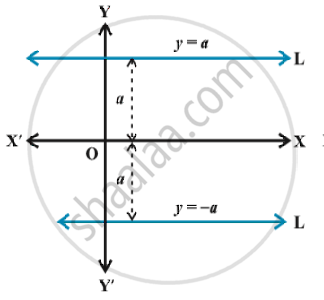Therefore, equation of the line L is either y =  a or y = – a. Choice of sign will depend upon the position of the line according as the line is above or below the y-axis. Similarly, the equation of a vertical line at a distance b from the y-axis is either x = b or x =  – b.Fig.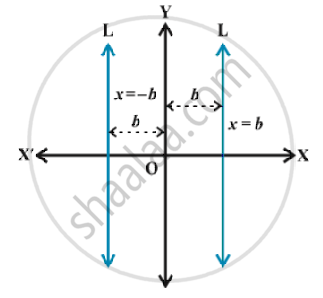Point-slope form:
Suppose that P_0 (x_0, y_0) is a fixed point on a non-vertical line L, whose slope is m. Let P (x, y) be an arbitrary point on L. fig.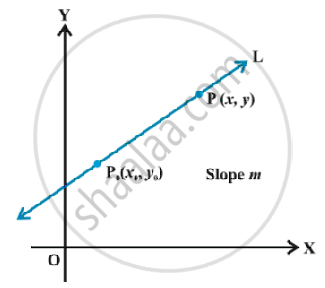Then, by the definition, the slope of L is given by
m = (y -y_0)/(x - x_0) ,i.e., y-y_0 = m(x-x_0)  ...(1)
Since the point P_0 (x_0 , y_0) along with all points (x, y) on L satisfies (1) and no other point in the plane satisfies (1). Equation (1) is indeed the equation for the given line L. Thus, the point (x, y) lies on the line with slope m through the fixed point (x_0, y_0), if and only if, its coordinates satisfy the equation   y – y_0 = m (x – x_0)

Two-point form :
Let the line L passes through two given points P_1 (x_1, y_1) and P_2 (x_2, y_2). Let P (x, y) be a general point on L .Fig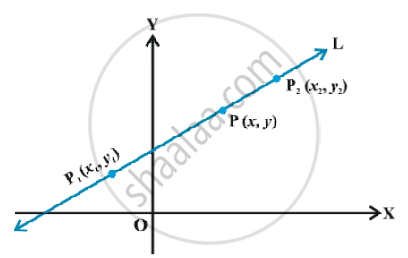The three points P_1, P_2 and P are collinear, therefore, we have slope of P_1 P = slope of P_1P_2.

i.e., (y-y_1)/(x -x_1)=(y_2-y_1)/(x_2-x_1) or

y -y_1 = (y_2-y_1)/(x_2-x_1)(x-x_1).
Thus, equation of the line passing through the points (x_1, y_1) and (x_2, y_2) is given by

y -y_1 = (y_2-y_1)/(x_2-x_1)(x-x_1)     ....(2)

Slope-intercept form:

Suppose a line L with slope m cuts the y-axis at a distance c from the origin Fig.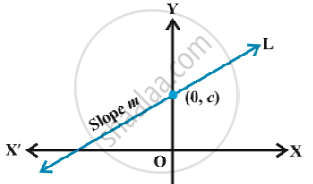The distance c is called the yintercept of the line L. Obviously, coordinates of the point where the line meet the y-axis are
(0, c). Thus, L has slope m and passes through a fixed point (0, c). Therefore, by point-slope form, the equation of L is
y-c =m(x-0)  or  y =mx + c
Thus, the point (x, y) on the line with slope m and y-intercept c lies on the line if and only if  y = mx +c    ...(3)
Note that the value of c will be positive or negative according as the intercept is made on the positive or negative side of the y-axis, respectively.

Intercept - form:
Suppose a line L makes x-intercept a and y-intercept b on the axes. Obviously L meets x-axis at the point (a, 0) and y-axis at the point (0, b).Fig.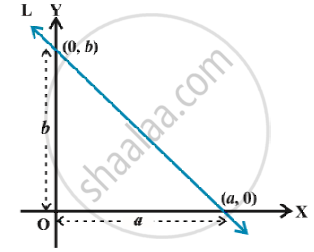By two-point form of the equation of the line, we have
y - 0 =(b-0)/(0-a)(x - a) or ay = -bx +ab ,

i.e., x/a+y/b=1.

Normal  form :
Suppose a non-vertical line is known to us with following data:
(i) Length of the perpendicular (normal) from origin to the line.
(ii) Angle which normal makes with the positive direction of x-axis.
Let L be the line, whose perpendicular distance from origin O be OA = p and the angle between the positive x-axis and OA be ∠XOA = ω. The possible positions of line L in the Cartesian plane are shown in the fig. Now, our purpose is to find slope of L and a point on it.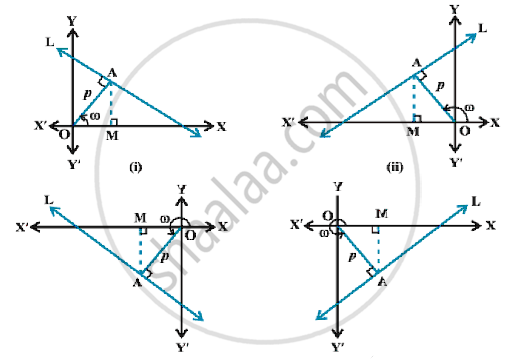Alternate method:

The simplest form of the equation of a line is y = mx + c.
Again assuming F along x-axis and K along  y-axis, we can take equation in the form
K = mF + c                   ... (1)
Equation (1) is satisfied by (32, 273) and (212, 373).
Therefore 273 = 32m + c                              ... (2)
and 373 = 212m + c                                       ... (3)
Solving (2) and (3), we get
m = 5/9 and c =2297/9.
Putting the values of m and c in (1) ,we get
K =5/9 F + 2297/9
which is the required relation.K=0,(4) gives
F = -459.4.

If you would like to contribute notes or other learning material, please submit them using the button below.

### Shaalaa.com

Various Forms of the Equation of a Line -horizontal and vertical lines [00:04:20]
S
0%